Download PDF文章快速检索 高级检索

The algebraic structure of rough linear approximation space
LIU Yamei, MA Yingcang, LU Wenxia, CHEN Yanyan
School of Science,Xi'an Polytechnic University,Xi'an 710048,China
Abstract: Focusing on the description of the algebraic structure on the upper(lower)approximation,and according to the properties of the upper(lower)approximation in rough linear space,two new sets are presented,and the inclusion relation of the upper approximation's union and the lower approximation's intersection are improved,deriving the equation expressions of the upper approximation's union and the lower approximation's intersection. Moreover,the rough linear approximation space is proposed and the intersection,union and complementary operations are introduced in the rough linear approximation space. Finally,it has been proven that the rough linear approximation space is Boolean algebra on the intersection,union and complementary operations. This paper enriches the combination of linear space and rough set research.
Key words: rough linear approximation space     linear subspace     congruence     upper and lower approximation     Boolean algebra     algebraic structure

1 基本概念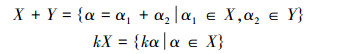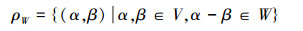1)ρWV上的一个同余关系

2)∀αV，同余类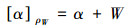则可将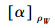记为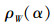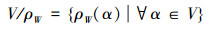是全体同余类的集合。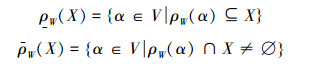1)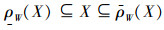2)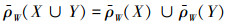3)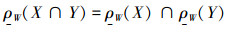4)若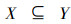，则有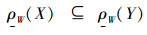,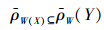5)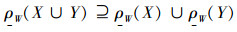6)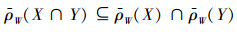2 集合的交(并)的上(下)近似的等式刻画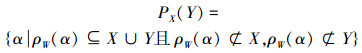;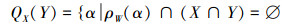,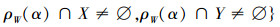1)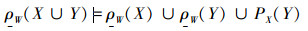2)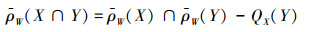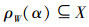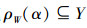，则有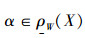,或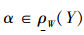，则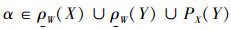。若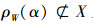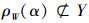，则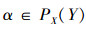，所以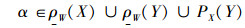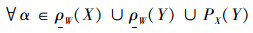，有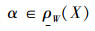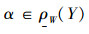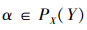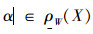，则有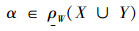，若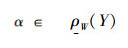，则有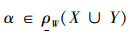，若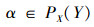，则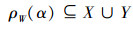，则有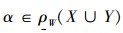2)此命题等价为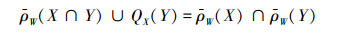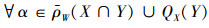，有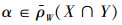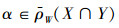，则有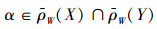，若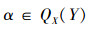，则有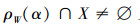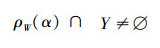，所以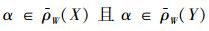，则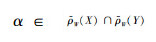。对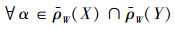，则有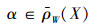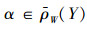，即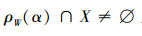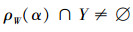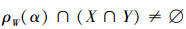，则有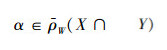；若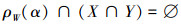，则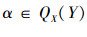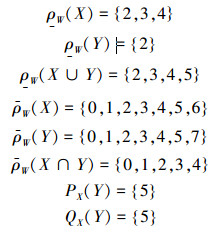3 粗糙线性近似空间及其代数结构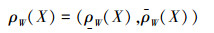1)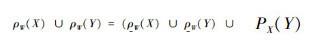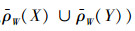2)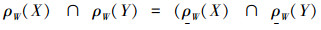,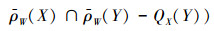3)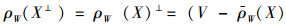,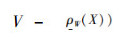4)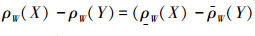,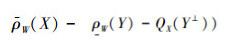2)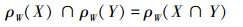1)交换律：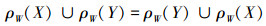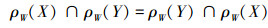2)结合律：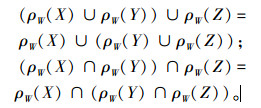3)分配律：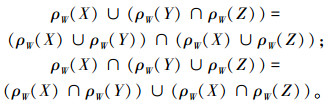4)幂等律：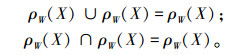5)0-1律：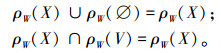6)互补律：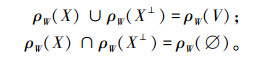7)对偶律：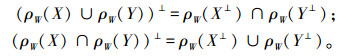1)由PX(Y)和QX(Y)定义可以看出PX(Y)=PY(X)，QX(Y)=QY(X)。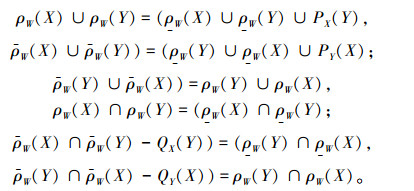2)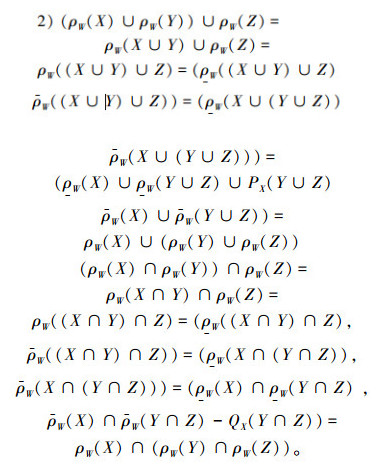3)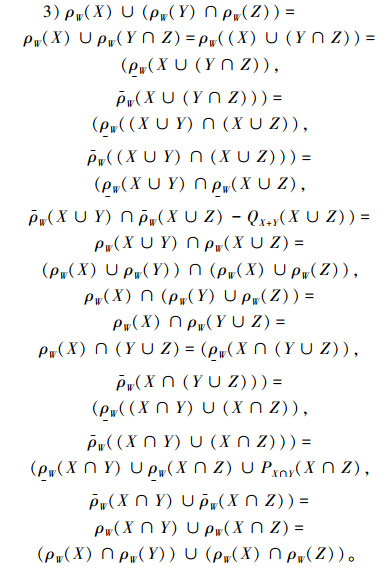4)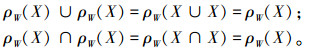5)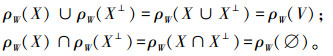6)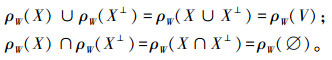7)要证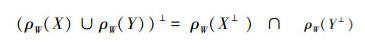，只需证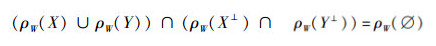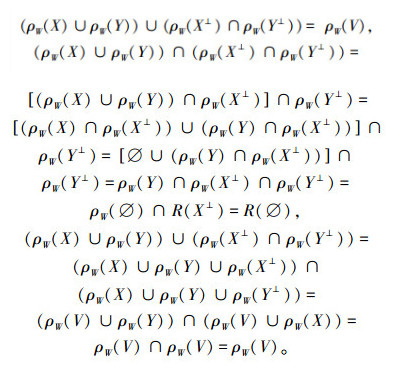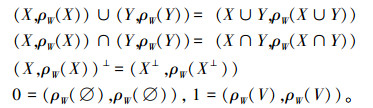4 结束语

  PAWLAK Z. Rough sets[J]. International Journal of Computer and Information Science , 1982, 11 : 341-356 DOI:10.1007/BF01001956  WU W Z, MI J S, ZHANG W X. Generalized fuzzy rough sets[J]. Information Sciences , 2003, 151 : 263-282 DOI:10.1016/S0020-0255(02)00379-1  WU W Z, ZHANG W X. Constructive and axiomatic approaches of fuzzy approximation operators[J]. Information Sciences , 2004, 159 : 233-254 DOI:10.1016/j.ins.2003.08.005  吴伟志, 张文修, 徐宗本. 粗糙模糊集的构造与公理化方法[J]. 计算机学报 , 2004, 27 (4) : 197-203 WU Zhiwei, ZHANG Wenxiu, XU Zongben. Characterizating rough fuzzy sets in constructive and axiomatic approaches[J]. Chinese Journal of Computers , 2004, 27 (4) : 197-203  YAO Y Y, LIN T Y. Generalization of rough sets using modal logic[J]. Intelligent Automation and Soft Computing: An International Journal , 1996, 2 : 103-120 DOI:10.1080/10798587.1996.10750660  MI J S, ZHANG W X. An axiomatic characterization of fuzzy generalization of rough sets[J]. International Journal of General Systems , 2005, 34 : 77-90 DOI:10.1080/03081070512331318329  YAO Y Y. Two views of the theory of rough sets in finite universes[J]. International Journal of Approximate Reasoning , 1996, 15 : 291-317 DOI:10.1016/S0888-613X(96)00071-0  YAO Y Y. Constructive and algebraic methods of the theory of rough sets[J]. Journal of Information Sciences , 1998, 109 : 21-47 DOI:10.1016/S0020-0255(98)00012-7  祝峰, 何华灿. 粗集的公理化[J]. 计算机学报 , 2000, 33 (3) : 330-333 ZHU Feng, HE Huacan. The axiomatization of the rough set[J]. Chinese Journal of Computers , 2000, 33 (3) : 330-333  韩素青. 粗糙群的同态与同构[J]. 山西大学学报:自然科学版 , 2001, 24 (4) : 303-305 HAN Suqing. Homomorphism and isomorphism of rough group[J]. Journal of Shanxi University :Natural Science Edition , 2001, 24 (4) : 303-305  张金玲, 张振良. 粗糙子群和粗糙子环[J]. 纯粹数学与应用数学 , 2004, 20 (1) : 92-96  韩素青, 胡桂荣. 粗糙陪集、粗糙不变子群[J]. 计算机科学期刊 , 2001, 28 (suppl.) : 76-77 HAN Suqing, HU Guirong. Rough coset and rough invariant subgroup[J]. Scientific Journal of Computer Science , 2001, 28 (suppl.) : 76-77  王德松, 舒兰. 粗糙不变子群的若干性质与粗糙商群[J]. 模糊系统与数学 , 2004, 18 (4) : 49-53 WANG Desong, SHU Lan. Some properties of rough invariant subgroups and rough quotient groups[J]. Fuzzy Systems and Mathematics , 2004, 18 (4) : 49-53  KUROKI N. Rough sets and convex subsets in a linear space[J]. RIMS Kokyuroku , 1997, 985 : 42-47  LIU W J. Rough linear space[J]. Fuzzy Systems and Mathematics , 2007, 21 : 137-143  LIU W J, YANG P L. The Fuzzy Rough linear space[J]. Fuzzy Systems and Mathematics , 2006, 20 : 135-140  吴明芬. 线性空间上基于同余的上下近似[J]. 模糊系统与数学 , 2008, 22 (1) : 146-150 WU Mingfen. Upper and lower approximation based on congruences on linear spaces[J]. Fuzzy Systems and Mathematics , 2008, 22 (1) : 146-150  张小红. 模糊逻辑及其代数分析. 北京:科学出版社[M]. 2008 : 133 -134.  ZHANG H G, LIANG H L, LIU D R. Two new operators in rough set theory with applications to fuzzy sets[J]. Information Sciences , 2004 (166) : 147-165
DOI: 10.3969/j.issn.1673-4785.201307016

0

#### 文章信息

LIU Yamei, MA Yingcang, LU Wenxia, CHEN Yanyan

The algebraic structure of rough linear approximation space

CAAI Transactions on Intelligent Systems, 2014, 9(2): 224-228
http://dx.doi.org/10.3969/j.issn.1673-4785.201307016